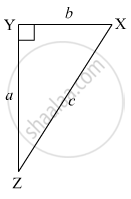# In the Right Angled δ Xyz, ∠ Xyz = 90° and A, B, C Are the Lengths of the Sides as Shown in the Figure. Write the Following Ratios, (I) Sin X (Ii) Tan Z (Iii) Cos X (Iv) Tan X. - Geometry

Sum

In the right angled triangle XYZ, angleXYZ = 90° and a, b, c are the lengths of the sides as shown in the figure. Write the following ratios,
(i) sin X (ii) tan Z (iii) cos X (iv) tan X.#### Solution(i) Sin X = "Opposite side of ∠ X"/"Hypotenuse" = ["YZ"]/["XZ"] = a/c

(ii) tan Z = "Opposite side of ∠ Z"/"Adjacent Side of ∠ Z" = ["XY"]/["YZ"] = b/a

(iii) Cos X = "Adjacent Side of ∠ Z"/"Hypotenuse" = ["XY"]/["XZ"] = b/c

(iv) tan X = " Opposite side of ∠X"/"Adjacent Side of ∠X" = "YZ"/"XY" = a/b

Concept: Terms related to right angled triangle
Is there an error in this question or solution?

#### APPEARS IN

Balbharati Mathematics 2 Geometry 9th Standard Maharashtra State Board
Chapter 8 Trigonometry
Practice Set 8.1 | Q 2 | Page 104